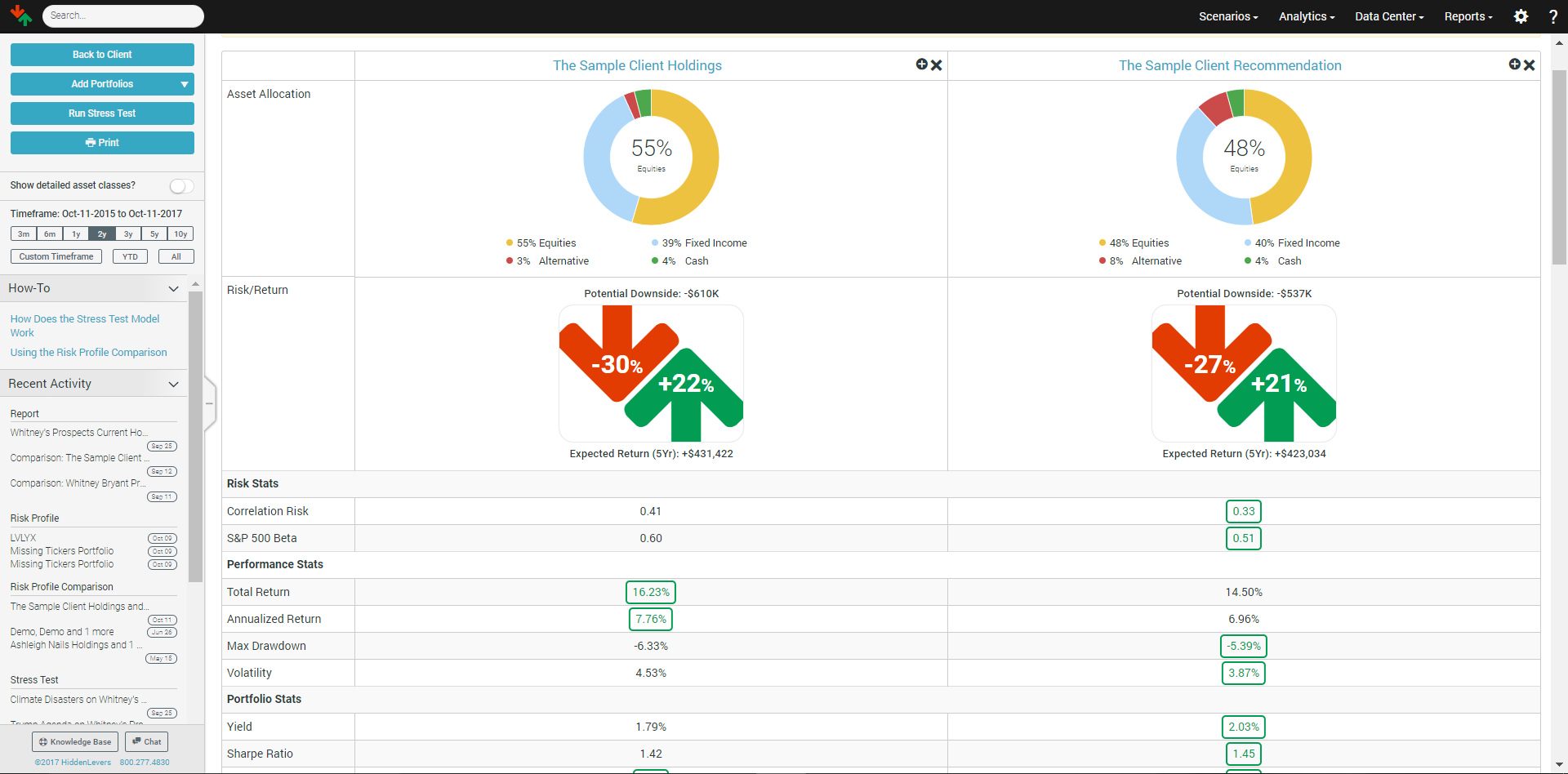The Risk Profile Comparison is a high-level risk overview for securities, portfolios, models, households, or any group of investments. It's a great interactive tool for you to compare + contrast your client's current holdings to your recommendation(s).

Below you will find descriptions of each modal in the Risk Profile Comparison; you can also find additional details by clicking the (+) in top right-hand corner of any section.1. Asset Allocation

The asset allocation for the portfolio is broken down into four major categories (Equity, Fixed Income, Alternative, and Cash), and a full listing of holdings is provided. The additional details screen shows specific characteristics of each holding.

2. Risk + Return

The number in the red arrow indicates the greatest potential loss across all of HiddenLevers' scenario outcomes. Conversely, the number in the green arrow indicates the greatest potential gain across all of HiddenLevers' scenario outcomes. The Additional Details popup gives a full listing of the potential upside and downside impact for all scenarios. To learn more about a topic and the specific outcomes, click the name of a scenario.  To get more information about how we calculate expected return click here

3. Risk Statistics

Correlation Risk - This number is a measure of both correlation and concentration risk, computed based on the portfolio's full correlation matrix. The metric ranges between 0 and 1, with 0 representing an infinite number of perfectly uncorrelated positions, and 1 representing any number of perfectly correlated positions. A single position portfolio also receives a score of 1 (based on extreme concentration risk).

Long-Term Beta -  Beta measures the relationship between an investment and a major market index. A beta of 1.0 means that a 1% rise in the S&P 500 could lead to a 1% rise in the investment, while a beta of -0.5 means that a 1% rise in the S&P 500 could lead to a 0.5% drop in the investment. The beta for an investment is determined by using regression analysis to measure the relationship between the returns of the investment and the returns of the S&P500. HiddenLevers uses performance history since 9/1/2008 for regression results. For investments without history since 9/1/2008, all available historical data is used.

4.Performance Statistics

Total Return - Calculation of returns of all securities inside of portfolio over the timeframe selected. The calculation assumes the same portfolio for entire timeframe rebalanced weekly. Yield and expense ratio for each security is included in this calculation.

Annualized Return - An annualized total return is the geometric average amount of money earned by an investment each year over a given time period. Annualized return is used because the amount of investment lost or gained in a given year is interdependent with the amount from the other years under consideration because of compounding.

Max Drawdown - The actual historical peak to trough performance in the timeframe of the portfolio you are looking at

Volatility - HiddenLevers measures volatility as the annualized standard deviation of an investment or portfolio, expressed in percentage terms. The standard deviation is calculated using weekly data points and is then annualized by multiplying by the square-root of 52 (number of periods in one year).

5. Portfolio Statistics

Yield -The weighted average of the current yield (data updated monthly) for securities in the portfolio.

Sharpe Ratio - The Sharpe ratio is the average return earned in excess of the risk-free rate per unit of volatility or total risk.

Sortino Ratio - The Sortino Ratio subtracts the risk-free rate of return from the portfolio's return and then divides that by the downside deviation.

6. Expenses

Expense Ratio - the weighted average of the most recently disclosed net expense ratio for securities in the portfolio

AUM Fee -  the AUM fee of each portfolio.

7. Stress Test Risk

This section of the Risk Profile Comparison shows the maximum downside of each portfolio using your default scenarios. You can see the maximum downside of each scenario by clicking "Show All Scenarios."

If you'd like to change your default scenarios go to "Model Settings" and click the "Default Scenarios" link.

8. Performance History

The chart shows the performance of each portfolio chosen against one another. The timeframe of this chart can be changed by using the "Timeframe" feature which can be found to the left of the Risk Profile Comparison.

Please note that this chart does not take into account trading history as HiddenLevers does not store the actual trading history for an account. The chart reflects the performance of a static portfolio.

9. Compare Positions

This section is often referred to as the "Transition Plan" as it compares the first portfolio against each portfolio you choose, and the amount allocated to each security.